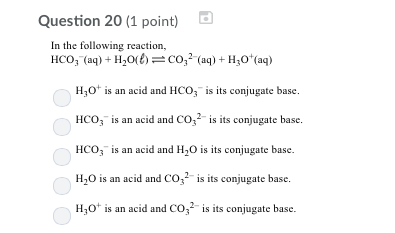# Question & Answer: In the following reaction, HCO_3^- (aq) + H_2O(l) rightleftharpoons CO_3^2- (aq) +…..In the following reaction, HCO_3^- (aq) + H_2O(l) rightleftharpoons CO_3^2- (aq) + H_3O^+ (aq) H_3O^+ is an acid and HCO_3^- is its conjugate base. HCO_3^- is an acid and CO_3^2- is its conjugate base. HCO_3^- is an acid and H_2O is its conjugate base. H_2O is an acid and CO_3^2- is its conjugate base. H_3O^+ is an acid and CO_3^2- is its conjugate base.